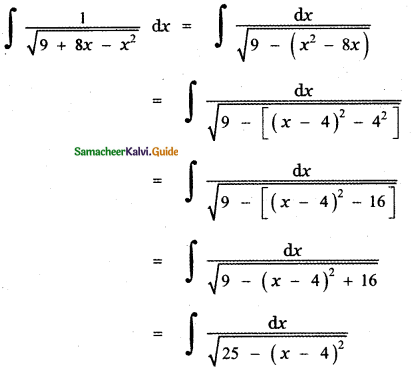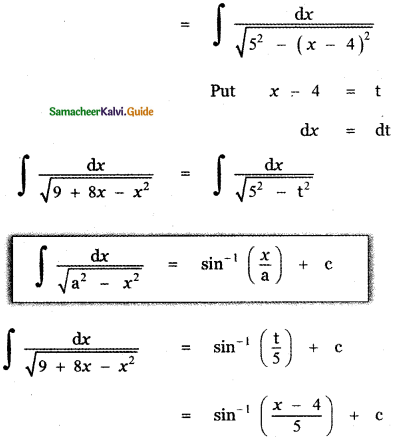Tamilnadu State Board New Syllabus Samacheer Kalvi 11th Maths Guide Pdf Chapter 11 Integral Calculus Ex 11.10 Text Book Back Questions and Answers, Notes.

## Tamilnadu Samacheer Kalvi 11th Maths Solutions Chapter 11 Integral Calculus Ex 11.10

Find the integrals of the following:

Question 1.
(i) $$\frac{1}{4-x^{2}}$$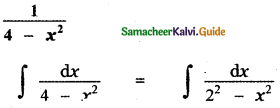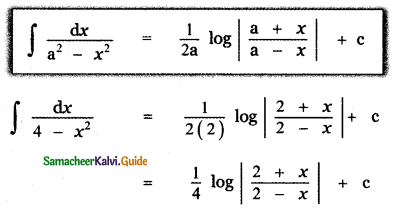(ii) $$\frac{1}{25-4 x^{2}}$$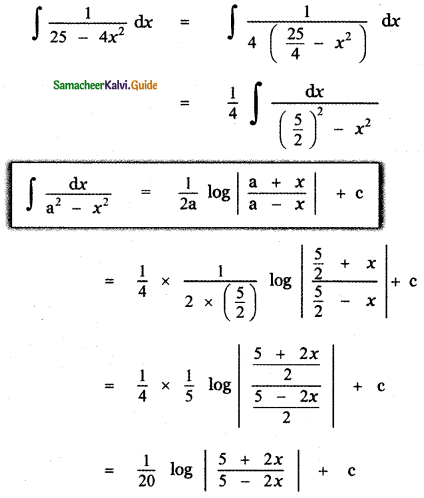(iii) $$\frac{1}{9 x^{2}-4}$$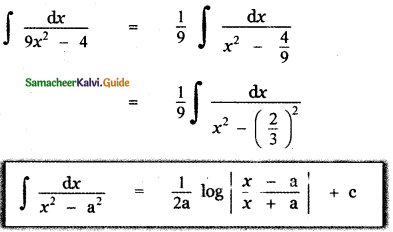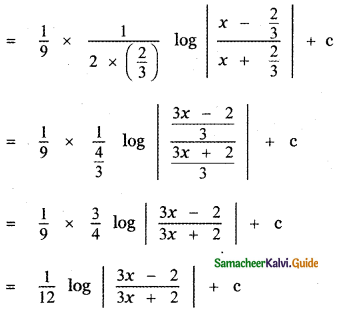Question 2.
(i) $$\frac{1}{6 x-7-x^{2}}$$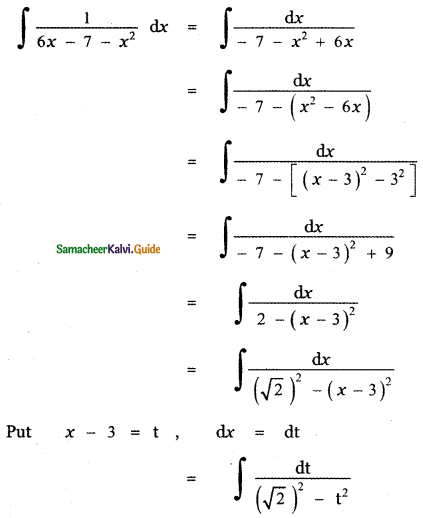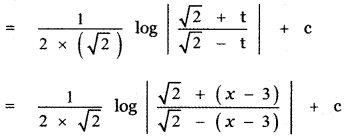(ii) $$\frac{1}{(x+1)^{2}-25}$$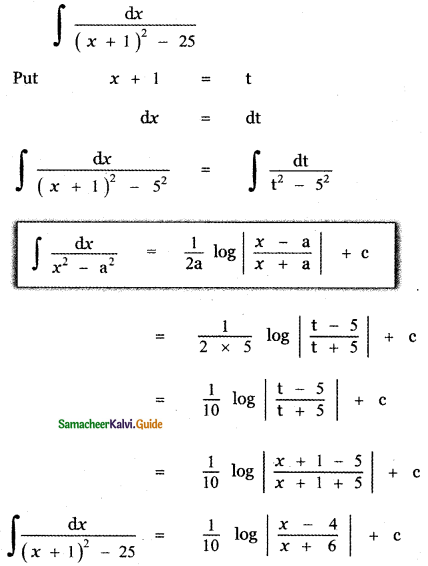(iii) $$\frac{1}{\sqrt{x^{2}+4 x+2}}$$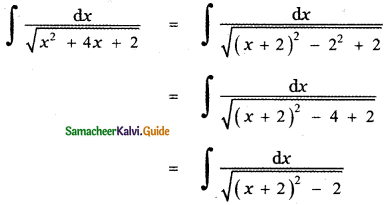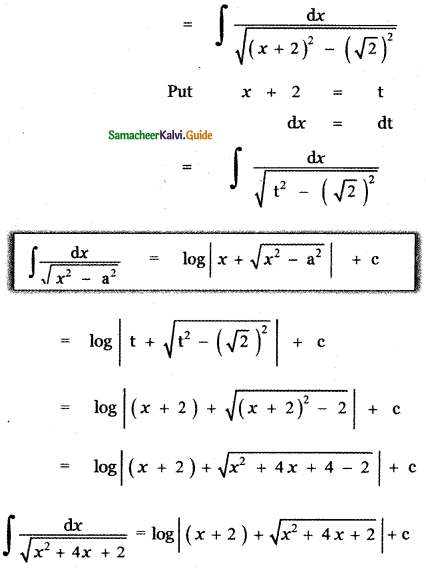Question 3.
(i) $$\frac{1}{\sqrt{(2+x)^{2}-1}}$$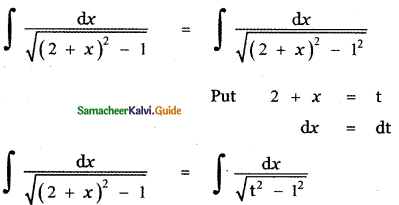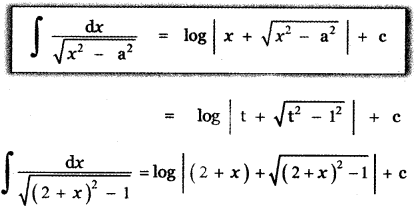(ii) $$\frac{1}{\sqrt{x^{2}-4 x+5}}$$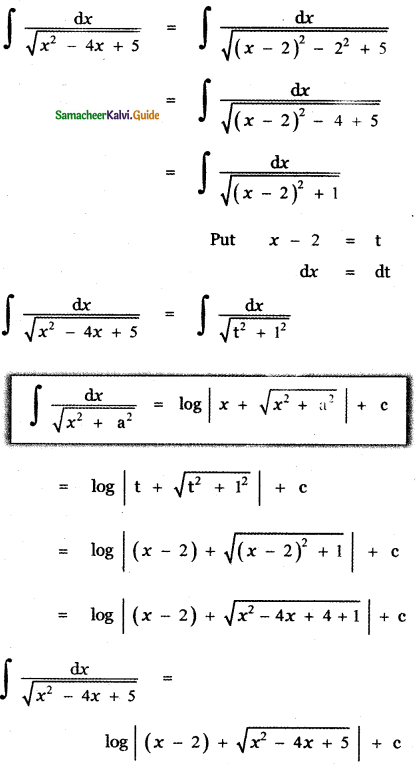(iii) $$\frac{1}{\sqrt{9+8 x-x^{2}}}$$